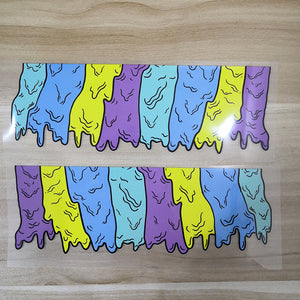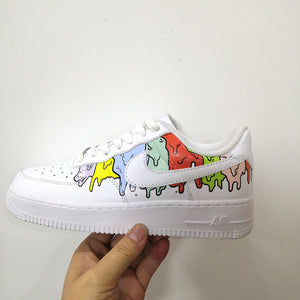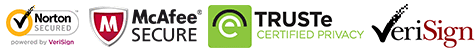Free shipping
Free shipping# Drip Patches For Custom Air Force 1s Drip, Easy Iron On Patches For DIY or Custom Shoes

Regular price \$15.00 \$9.90 Save \$5.10

ONLY 177759 LEFT

 Hours   Minutes   Seconds

Sale Ends Once The Timer Hits Zero!

ONLY 177759 LEFT

Get these easy use iron on honey drip patches to DIY to create your own drip shoes. Best size for DIY or custom  Air Force 1 & Vans.

<<<<<<<<<<<<<<<<<<<<<<<<<<<<<<<<<<<<<<<<<<<<<<<<<<<<<<<

Please STOP wasting money to buy the "NO RETURN, NO REFUND drip shoes. Try this iron on drip stickers to DIY. Save lots of money!

<<<<<<<<<<<<<<<<<<<<<<<<<<<<<<<<<<<<<<<<<<<<<<<<<<<<<<<

Why use these honey drip patches:

• 1. Perfect sizes for sneakers and other items, such as socks, hoodies, jeans, jackets....
• 2. Waterproof and flexible, when iron on, proved durable after washing test and brushing test. You can check the videos at the bottom of front page or my YouTube Channel: theshoesgirl
• 3. Easy use, just iron on directly, no need any transfer paper, no need any sealer. Will not peel off even brushing in water! You can watch the test in my videos.

<<<<<<<<<<<<<<<<<<<<<<<<<<<<<<<<<<<<<<<<<<<<<<<<<<<<<<<

• The set includes 2 sheets of honey drip patches, can make one pair of shoes
• The shoes sample is another design, this one had not made sample yet, they look similar.
• The shoes are not included in the set.

<<<<<<<<<<<<<<<<<<<<<<<<<<<<<<<<<<<<<<<<<<<<<<<<<<<<<<<
• ### Size:

• width 19 cm
• height: 6.5 cm

<<<<<<<<<<<<<<<<<<<<<<<<<<<<<<<<<<<<<<<<<<<<<<<<<<<<<<<

### How to use:

1. Position the drip patches on the shoes with tape

2. Iron on the top of the drip, please keep temperature 135-150℃

3. peel the vinyl, the rest of drip patches will stick on the shoes.

4. If use normal iron, please turn off the steam, keep the temperature above, use the tip and be careful and patient, for it's heavy to control, better use the mini iron.

5. Watch the tutorial video here:

the video is for another similar design, but the process is the same.

<<<<<<<<<<<<<<<<<<<<<<<<<<<<<<<<<<<<<<<<<<<<<<<<<<<<<<<

FREE SHIPPING! Ship within 1-2 days.

people are currently looking at this product

Estimated delivery time 7-15 days

Guaranteed Safe Checkout